# Solution assignment 04 Optimizing f(x)

### Assignment 4

Determine the extreme of the function: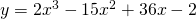and verify whether it is a maximum or a minimum by using the second derivative.

### Solution

In order to find the candidates of extremes we have to calculate the derivative of the function and solve the following equation: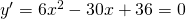thus: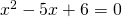and this equation has the solution: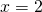or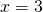The second derivative is: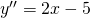Forthe second derivative is negative and thus there is a maximum for.
Forthe second derivative is positive and thus there is a minimum for.

0